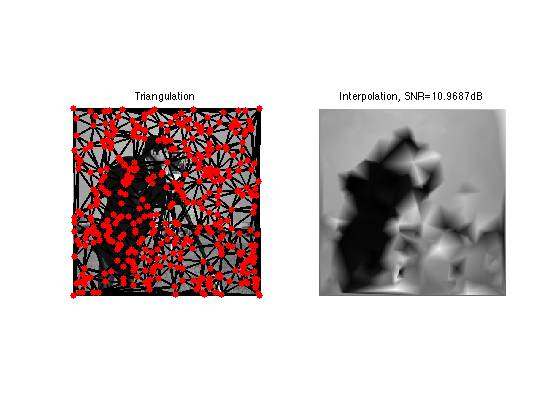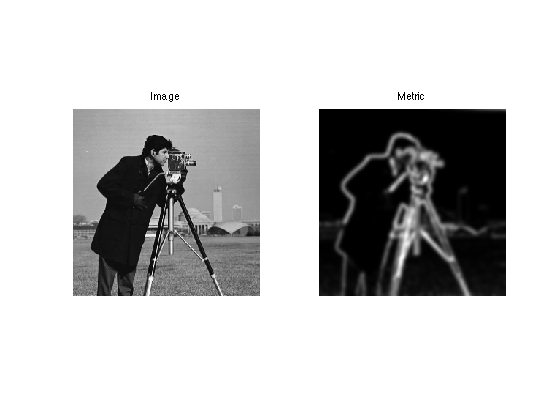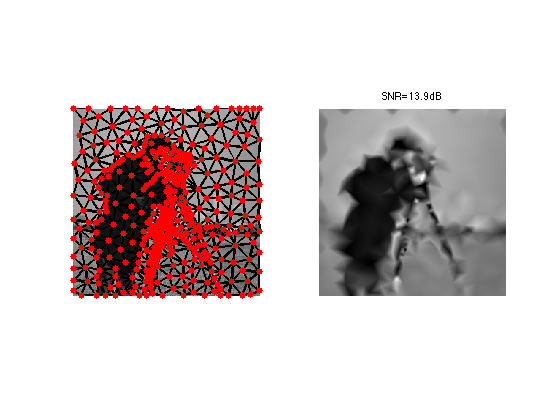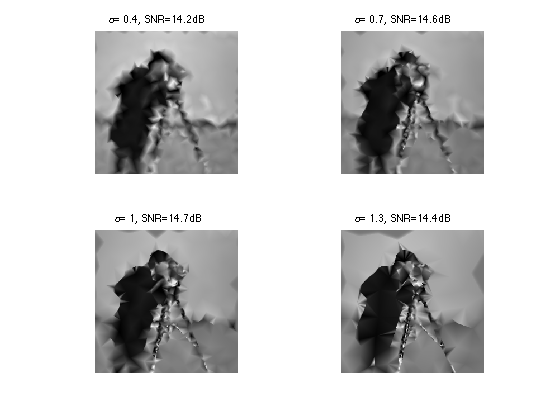$\newcommand{\NN}{\mathbb{N}} \newcommand{\CC}{\mathbb{C}} \newcommand{\GG}{\mathbb{G}} \newcommand{\LL}{\mathbb{L}} \newcommand{\PP}{\mathbb{P}} \newcommand{\QQ}{\mathbb{Q}} \newcommand{\RR}{\mathbb{R}} \newcommand{\VV}{\mathbb{V}} \newcommand{\ZZ}{\mathbb{Z}} \newcommand{\FF}{\mathbb{F}} \newcommand{\KK}{\mathbb{K}} \newcommand{\UU}{\mathbb{U}} \newcommand{\EE}{\mathbb{E}} \newcommand{\Aa}{\mathcal{A}} \newcommand{\Bb}{\mathcal{B}} \newcommand{\Cc}{\mathcal{C}} \newcommand{\Dd}{\mathcal{D}} \newcommand{\Ee}{\mathcal{E}} \newcommand{\Ff}{\mathcal{F}} \newcommand{\Gg}{\mathcal{G}} \newcommand{\Hh}{\mathcal{H}} \newcommand{\Ii}{\mathcal{I}} \newcommand{\Jj}{\mathcal{J}} \newcommand{\Kk}{\mathcal{K}} \newcommand{\Ll}{\mathcal{L}} \newcommand{\Mm}{\mathcal{M}} \newcommand{\Nn}{\mathcal{N}} \newcommand{\Oo}{\mathcal{O}} \newcommand{\Pp}{\mathcal{P}} \newcommand{\Qq}{\mathcal{Q}} \newcommand{\Rr}{\mathcal{R}} \newcommand{\Ss}{\mathcal{S}} \newcommand{\Tt}{\mathcal{T}} \newcommand{\Uu}{\mathcal{U}} \newcommand{\Vv}{\mathcal{V}} \newcommand{\Ww}{\mathcal{W}} \newcommand{\Xx}{\mathcal{X}} \newcommand{\Yy}{\mathcal{Y}} \newcommand{\Zz}{\mathcal{Z}} \newcommand{\al}{\alpha} \newcommand{\la}{\lambda} \newcommand{\ga}{\gamma} \newcommand{\Ga}{\Gamma} \newcommand{\La}{\Lambda} \newcommand{\Si}{\Sigma} \newcommand{\si}{\sigma} \newcommand{\be}{\beta} \newcommand{\de}{\delta} \newcommand{\De}{\Delta} \renewcommand{\phi}{\varphi} \renewcommand{\th}{\theta} \newcommand{\om}{\omega} \newcommand{\Om}{\Omega} \renewcommand{\epsilon}{\varepsilon} \newcommand{\Calpha}{\mathrm{C}^\al} \newcommand{\Cbeta}{\mathrm{C}^\be} \newcommand{\Cal}{\text{C}^\al} \newcommand{\Cdeux}{\text{C}^{2}} \newcommand{\Cun}{\text{C}^{1}} \newcommand{\Calt}{\text{C}^{#1}} \newcommand{\lun}{\ell^1} \newcommand{\ldeux}{\ell^2} \newcommand{\linf}{\ell^\infty} \newcommand{\ldeuxj}{{\ldeux_j}} \newcommand{\Lun}{\text{\upshape L}^1} \newcommand{\Ldeux}{\text{\upshape L}^2} \newcommand{\Lp}{\text{\upshape L}^p} \newcommand{\Lq}{\text{\upshape L}^q} \newcommand{\Linf}{\text{\upshape L}^\infty} \newcommand{\lzero}{\ell^0} \newcommand{\lp}{\ell^p} \renewcommand{\d}{\ins{d}} \newcommand{\Grad}{\text{Grad}} \newcommand{\grad}{\text{grad}} \renewcommand{\div}{\text{div}} \newcommand{\diag}{\text{diag}} \newcommand{\pd}{ \frac{ \partial #1}{\partial #2} } \newcommand{\pdd}{ \frac{ \partial^2 #1}{\partial #2^2} } \newcommand{\dotp}{\langle #1,\,#2\rangle} \newcommand{\norm}{|\!| #1 |\!|} \newcommand{\normi}{\norm{#1}_{\infty}} \newcommand{\normu}{\norm{#1}_{1}} \newcommand{\normz}{\norm{#1}_{0}} \newcommand{\abs}{\vert #1 \vert} \newcommand{\argmin}{\text{argmin}} \newcommand{\argmax}{\text{argmax}} \newcommand{\uargmin}{\underset{#1}{\argmin}\;} \newcommand{\uargmax}{\underset{#1}{\argmax}\;} \newcommand{\umin}{\underset{#1}{\min}\;} \newcommand{\umax}{\underset{#1}{\max}\;} \newcommand{\pa}{\left( #1 \right)} \newcommand{\choice}{ \left\{ \begin{array}{l} #1 \end{array} \right. } \newcommand{\enscond}{ \left\{ #1 \;:\; #2 \right\} } \newcommand{\qandq}{ \quad \text{and} \quad } \newcommand{\qqandqq}{ \qquad \text{and} \qquad } \newcommand{\qifq}{ \quad \text{if} \quad } \newcommand{\qqifqq}{ \qquad \text{if} \qquad } \newcommand{\qwhereq}{ \quad \text{where} \quad } \newcommand{\qqwhereqq}{ \qquad \text{where} \qquad } \newcommand{\qwithq}{ \quad \text{with} \quad } \newcommand{\qqwithqq}{ \qquad \text{with} \qquad } \newcommand{\qforq}{ \quad \text{for} \quad } \newcommand{\qqforqq}{ \qquad \text{for} \qquad } \newcommand{\qqsinceqq}{ \qquad \text{since} \qquad } \newcommand{\qsinceq}{ \quad \text{since} \quad } \newcommand{\qarrq}{\quad\Longrightarrow\quad} \newcommand{\qqarrqq}{\quad\Longrightarrow\quad} \newcommand{\qiffq}{\quad\Longleftrightarrow\quad} \newcommand{\qqiffqq}{\qquad\Longleftrightarrow\qquad} \newcommand{\qsubjq}{ \quad \text{subject to} \quad } \newcommand{\qqsubjqq}{ \qquad \text{subject to} \qquad }$

Geodesic Triangulation for Image Compression

# Geodesic Triangulation for Image Compression

This tour explores the use geodesic triangulations to perform image compression.

## Installing toolboxes and setting up the path.

You need to download the following files: signal toolbox, general toolbox and graph toolbox.

You need to unzip these toolboxes in your working directory, so that you have toolbox_signal, toolbox_general and toolbox_graph in your directory.

For Scilab user: you must replace the Matlab comment '%' by its Scilab counterpart '//'.

Recommandation: You should create a text file named for instance numericaltour.sce (in Scilab) or numericaltour.m (in Matlab) to write all the Scilab/Matlab command you want to execute. Then, simply run exec('numericaltour.sce'); (in Scilab) or numericaltour; (in Matlab) to run the commands.

Execute this line only if you are using Matlab.

getd = @(p)path(p,path); % scilab users must *not* execute this


Then you can add the toolboxes to the path.

getd('toolbox_signal/');
getd('toolbox_general/');
getd('toolbox_graph/');


## Image Approximation with Triangulation

It is possible to approximate an image over a triangulation using piecewise linear splines.

Load an image.

name = 'cameraman';
n = 256;
M = rescale( load_image(name, n) );


Number of points used to compute the approximation. The more points, the smallest the error.

m = 400;


Seeds random points, include the corners into these points.

vertex = floor( rand(2,m-4)*(n-1) ) +1;
vertex(:,end+1:end+4) = [[1;1] [1;n] [n;n] [n;1]];


Compute a Delaunay triangulation.

faces = compute_delaunay(vertex);


A first way to perform an approximation with m triangles is to interpolate the image at the sampling points vertex.

vinterp = interp2(M, vertex(2,:), vertex(1,:));


Each vinterp(i) is the value of the approximating image at the vertex(:,i). We compute a spline interpolation.

Minterp = compute_triangulation_interpolation(faces,vertex,vinterp, n);


Display the approximation.

clf;
subplot(1,2,1);
plot_triangulation(vertex,faces, M);
title('Triangulation');
subplot(1,2,2);
imageplot(clamp(Minterp), ['Interpolation, SNR=' num2str(snr(Minterp,M)) 'dB']);Another, better way to compute the approximation is to compute coefficients vapprox that performs the best L2 approximation with linear spline.

vapprox = compute_orthoproj_triangulation(vertex, faces, M);
Mapprox = compute_triangulation_interpolation(faces,vertex,vapprox, n);


Compare interpolation and approximation.

clf;
imageplot(clamp(Minterp), ['Interpolation, SNR=' num2str(snr(Minterp,M),3) 'dB'], 1,2,1);
imageplot(clamp(Mapprox), ['Approximation, SNR=' num2str(snr(Mapprox,M),3) 'dB'], 1,2,2);## Isotropic Metrics for Image Approximation

It is possible to compute optimized sampling location vertex by using the farthest point sampling algorithm with a well chosen metric W so that more points are put in areas of strong gradient.

The metric will be of the form

W(x) = (norm(grad_x(M))+epsilon)^alpha|

where epsilon and alpha control the density variation strength.

Parameters for the metric.

alpha = .7;
epsilon = 1e-2;


Exercice 1: (check the solution) Compute a density function that is larger at area of large gradient. W(x) = (norm(grad(M))+epsilon)^alpha|, for alpha=.7. To stabilize the process, you can smooth a bit the gradient magnitude.

exo1;Exercice 2: (check the solution) Perform farthest points sampling to compute sampling location vertex and the corresponding geodesic Delaunay triangulation faces.

exo2;Perform approximation over the triangulation.

vgeod = compute_orthoproj_triangulation(vertex, faces, M);
Mgeod = compute_triangulation_interpolation(faces,vertex,vgeod, n);


Compare interpolation and approximation.

clf;
subplot(1,2,1);
plot_triangulation(vertex,faces, M);
subplot(1,2,2);
imageplot(clamp(Mgeod), ['SNR=' num2str(snr(Mgeod,M),3) 'dB']);Exercice 3: (check the solution) For a large value of m compute the approximation for several alpha.

exo3;